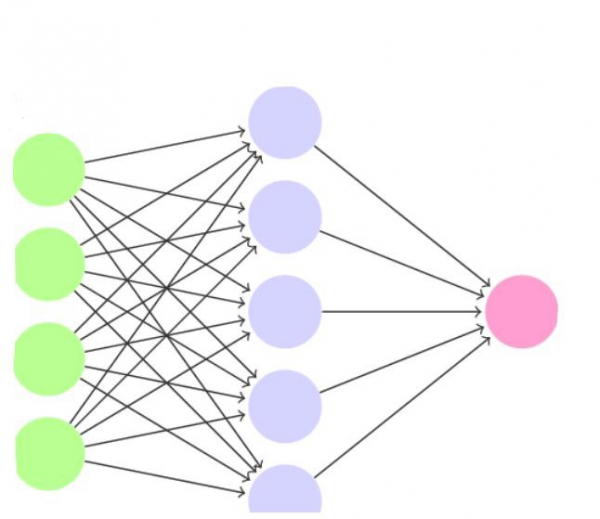# What are layers in a Neural Network with respect to Deep Learning in Machine Learning?

A neural network can contains any number of neurons. These neurons are organized in the form of interconnected layers. The input layer can be used to represent the dataset and the initial conditions on the data.

For example, suppose the input is a grayscale image, the output of every neuron in the input layer would be the intensity of every pixel of the image.

This is the reason we don’t count the input layer as a part of the other layers in the neural network. When we refer to a 1-layer net, we actually refer to a simple network that contains one single layer, the output, and the additional input layer.

We have previously seen that output layer can have one neuron. But there are cases where the output layer can have more than one neuron as well.

The case of output layer having more than one neuron is useful in classification, because each output neuron would represent one class.

Consider the example of the Modified National Institute of Standards and Technology (MNIST) dataset.

We can output multiple (10 in this case) neurons, where every neuron corresponds to one digit that belongs to any number between 0 and 0.

This way, the 1-layer neural network can also be used to classify the digit on each image.

This can be done by taking the output neuron that has the highest activation function value. If the highest activation function value is on y5 , we can understand that the network interprets the image shown as number 5.

The neurons of one-layer in the neural network can be connected to the neurons of other layers, but they can’t be connected to other neurons of the same layer.

## What is the need to organize the neurons in layers in the first place?

One reason is that the neuron conveys limited information (just a single value). But when the neurons in the layers are combined, their outputs produce a vector. Instead of single activation, the entire vector can now be considered. This way, a lot more information can be conveyed. This is because the vector contains multiple values, and the relative ratio between the values in the vector carry metadata.